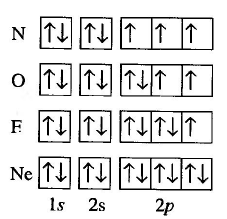The electronic configuration of an atom tells us how the electrons are distributed in the different layers, subshells and orbitals. This distribution is related to the physical properties of the elements. To write the electronic configuration of a polyelectronic atom we will use three rules: 1/ Filling or aufbau rule; 2/ Pauli exclusion principle; 3/ Hund's rule of maximum multiplicity.

• Rule of filling or aufbau. The electrons occupy the orbitals in such a way that the energy of the atom is minimized. That is, the lowest energy levels are occupied first. The order of filling the different sublayers is obtained by means of the following diagram in which we must start with the upper line and follow the arrows.• Pauli exclusion principle. Two electrons in an atom cannot have the same four quantum numbers. The quantum numbers n, and m determine an orbital in which only two electrons with different values of $m_s$ can enter.
• Hund's rule of maximum multiplicity tells us that orbitals of equal energy are filled with an electron (half-filled), once half-occupied the filling begins.

In the following image we can see the application of the Pauli principle and Hund's rule to the filling of orbitals for nitrogen, oxygen, fluorine and neon.Now we are going to write the electronic configuration of some atoms based on the previous rules:

H: $1s^1$

He: $1s^2$

Li: $1s^22s^1$

Be: $1s^22s^2$

B: $1s^22s^22p^1$

C:$1s^22s^22p^2$

N: $1s^22s^22p^3$

Or: $1s^22s^22p^4$

F: $1s^22s^22p^5$

Ne: $1s^22s^22p^6$

Na: Ne: $1s^22s^22p^63s^1$

Mg: $1s^22s^22p^63s^2$

At: $1s^22s^22p^63s^23p^1$

Yes: $1s^22s^22p^63s^23p^2$

Q: $1s^22s^22p^63s^23p^3$

S: $1s^22s^22p^63s^23p^4$

Cl: $1s^22s^22p^63s^23p^5$

Ar: $1s^22s^22p^63s^23p^6$